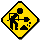This page is still under construction. The black background with red and blue lines maybe hard for some people see. Sometime soon I'll use a more user-friendly colour scheme. The thumbnails don't really do the images justice. To get the best view I recommend clicking on the images.

# Prime Numbers

The images on this page have been produced by performing a mod 5 on the difference between consecutive prime numbers. So, for example, the numbers 13 and 17 are consecutive primes and the difference between the 2 is 4. A mod 5 is then performed on the result of 4, which just happens to be 4 (4 mod 5 = 4). The result of the mod operation then moves a pen either up, down, left or right (1 pixel at a time). Unlike the prime number islands, the result of this mod operation has 5 possibilities (0, 1, 2, 3, or 4). As the pen can only move in 4 directions, one of the mod 5 outcomes is ignored, which results in the pen not moving for that answer. At some point in the future I'll put the executable and source code on this page. Feel free to send any questions or comments to craig@craigsarea.com

I've put some example images below. The left hand column of images represents the results of a mod 5 while only using an answer of 0 (UP), 1 (RIGHT), 2 (DOWN), or 3 (LEFT) to move the pen (an answer of 4 is ignored). The right hand column performs the same mod 5 on the same prime numbers, but only answers of 1 (UP), 2 (RIGHT), 3 (DOWN), or 4 (LEFT) move the pen (an answer of 0 is ignored). Click on any of the images to view the full size JPEG image. The full size images have a large width and height, but the filesizes are small (less than 200K). The JPEG compression causes some data loss in the full size images, but it still gives a good representation of the results. Over the next few days I'll upload the original zipped up BMP files.

I was suprised at how straight and consistant the lines are. Also, no matter what range of primes I use, the lines always seem to follow the same trend (angle, thickness and direction). Interestingly, in most (hundreds) of the images I have generated the red and blue lines grow close to each other, touch, and then grow apart.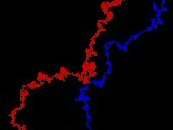Sometimes the lines touch and follow each other before growing apart. They seem to rarely cross each other. On the ocasions they do cross each other, they seem to cross back quite quickly. The lines also seem to have a fairly consistant thickness. The difference between 2 consecutive prime numbers can only move the pen a single pixel at a time. All the images I have generated seem to have lines several pixels thick. The left hand images, which ignore a mod 5 answer of 4, seem quite similar to the right hand images, which ignore a mod 5 answer of 0, but the left hand images always seem to be longer. The reason for the rotation between the left hand and right images is merely due to the way I have plotted them and is not really a factor of the prime numbers. In some parts of the images the lines seem to almost mirror each other.

Click on the thumbnails to see the full size image.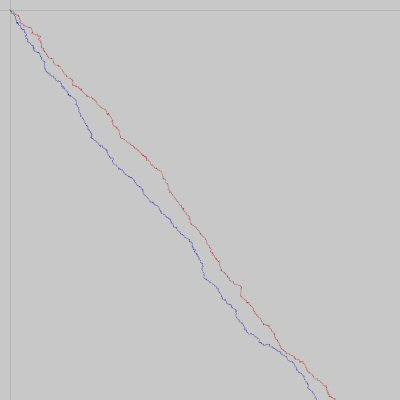Red = All the prime numbers between 10 * 106 and 11 * 106 Blue = All the prime numbers between 11 * 106 and 12 * 106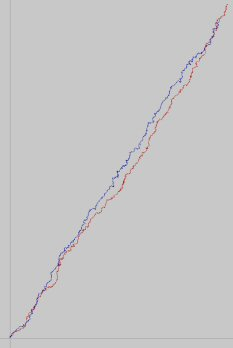Red = All the prime numbers between 20 * 106 and 21 * 106 Blue = All the prime numbers between 21 * 106 and 22 * 106Red = All the prime numbers between 30 * 106 and 31 * 106 Blue = All the prime numbers between 31 * 106 and 32 * 106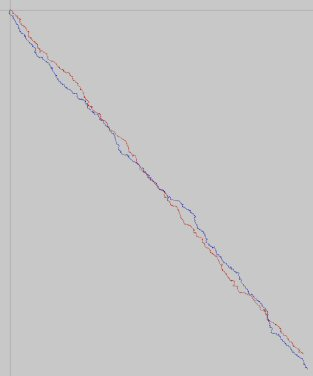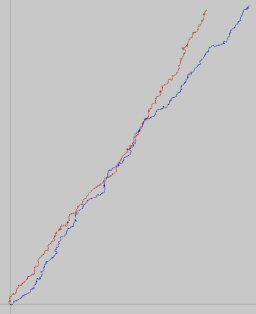Red = All the prime numbers between 40 * 106 and 41 * 106 Blue = All the prime numbers between 41 * 106 and 42 * 106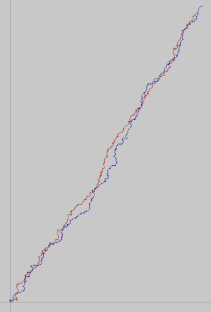Red = All the prime numbers between 50 * 106 and 51 * 106 Blue = All the prime numbers between 51 * 106 and 52 * 106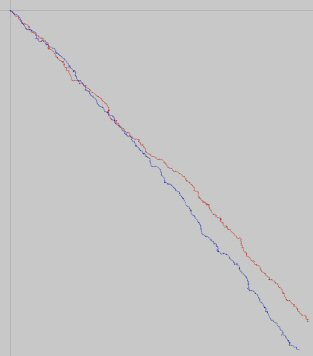Red = All the prime numbers between 60 * 106 and 61 * 106 Blue = All the prime numbers between 61 * 106 and 62 * 106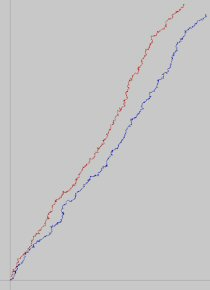Red = All the prime numbers between 70 * 106 and 71 * 106 Blue = All the prime numbers between 71 * 106 and 72 * 106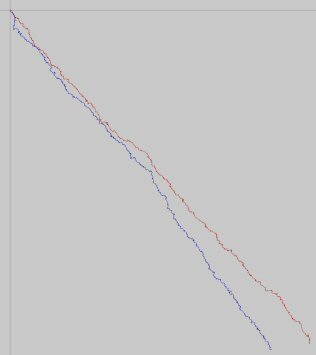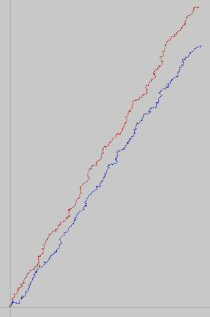Red = All the prime numbers between 80 * 106 and 81 * 106 Blue = All the prime numbers between 81 * 106 and 82 * 106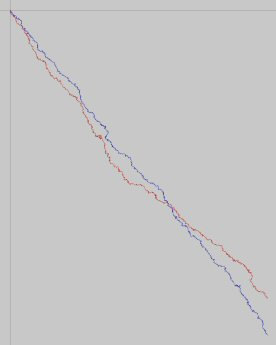Red = All the prime numbers between 90 * 106 and 91 * 106 Blue = All the prime numbers between 91 * 106 and 92 * 106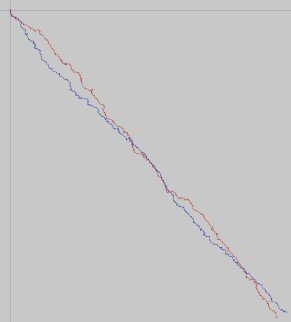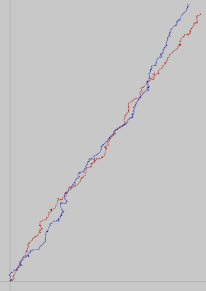Red = All the prime numbers between 100 * 106 and 101 * 106 Blue = All the prime numbers between 101 * 106 and 102 * 106
More to come soon.